Abstract: 本文主要介绍集合论中集合的基本运算和运算法则
Keywords: Complement，Union，Intersection，Commutative Law，Associative Law，Distributive laws

# 集合操作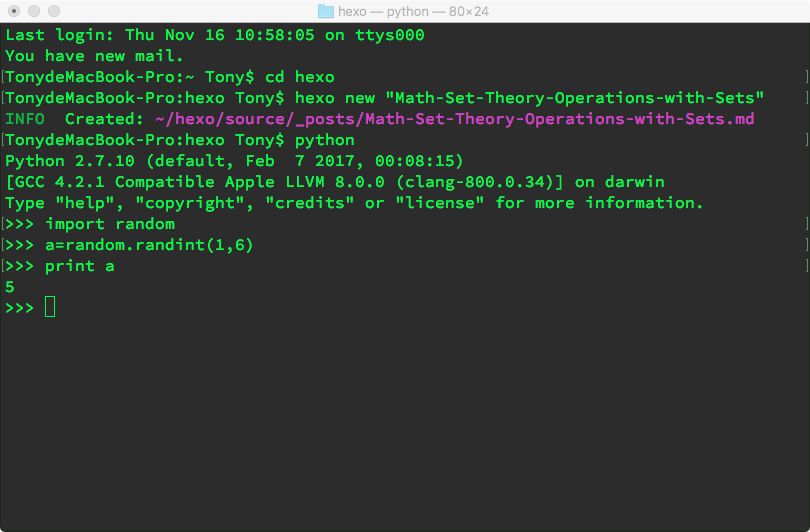1到6 ，结果是5

## Operation

### Complement

$$A^c={\omega | \omega \notin A}$$
$A^c$ 表示在空间 $\Omega$ 中不属于 $A$ 的元素的集合

1. $\Omega^c=\emptyset$
2. $\emptyset^c=\Omega$
3. $(A^c)^c=A$

### Union

$$A \cup B={\omega \in A \, or\, \omega \in B}$$

### Intersection

$$A \cap B={\omega \in A \, and\, \omega \in B}$$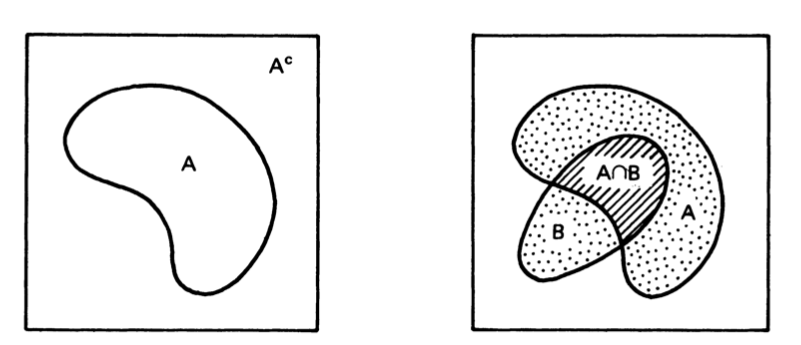### Commutative Law & Associative Law

$$A \cup B=B \cup A\\ A \cap B=B \cap A$$

$$(A \cup B)\cup C= A \cup (B \cup C)\\ (A \cap B)\cap C= A \cap (B \cap C)$$

$$\cup \leftrightarrow +\\ \cap \leftrightarrow \times$$

$$(A \cup B )\cap C \neq A \cup (B \cap C)$$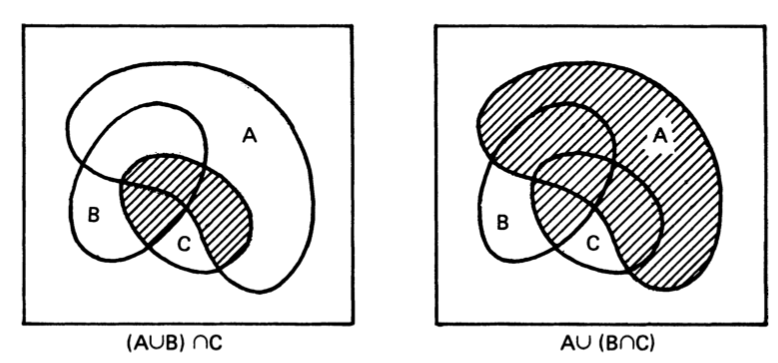Venn图怎么画？画圈就行了，相交的地方是交集，合起来是并集，没圈到的地方是complement，这个我看五年级的小孩都在奥数题里面写这个。

### Distributive Law

$$(A \cup B) \cap C=(A \cap C) \cup (B \cap C) \dots\dots (D_1)\\ (A \cap B) \cup C=(A \cup C) \cap (B \cup C) \dots\dots (D_2)$$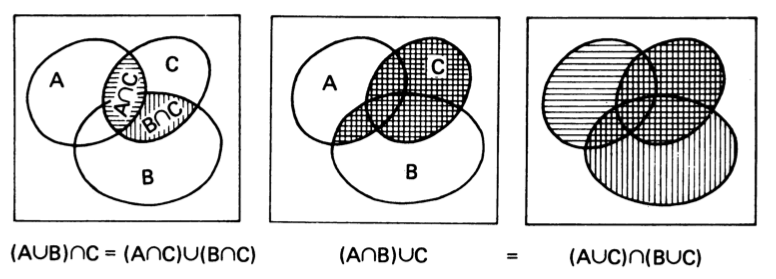##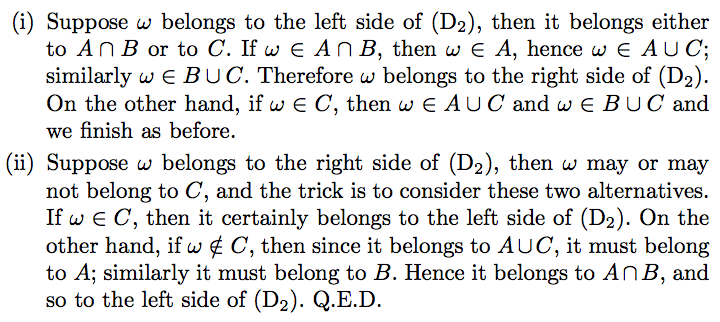I:假设元素属于左边，那么它属于A交B或者属于C。
①如果属于A交B那么 肯定属于A并C也要属于B并C，所以属于右边
$$suppos:\\ \omega \in A \cap B\\ then:\\ \omega \in A \cup C\\ \omega \in B \cup C$$
②如果属于C那么肯定也属于右边了
$$suppos:\\ \omega \in C\\ then:\\ \omega \in A \cup C\\ \omega \in B \cup C$$
II假设元素属于右边
①如果元素属于C，那么肯定属于左边
$$suppos:\\ \omega \in C\\ then:\\ \omega \in (A \cap B) \cup C$$
②如果元素不属于C，属于A并C同时也属于B并C，那么元素属于A并且属于B，所以元素属于A并B属于左边
$$suppos:\\ \omega \in A \cup C\\ \omega \in B \cup C\\ \omega \notin C\\ then:\\ \omega \in A \\ \omega \in B \\ \omega \in A \cap B \\$$
Q.E.D

0%